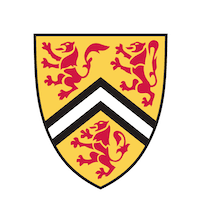# POTW：指数是甚？

170 0

前段时间由于 mocks 的种种原因从 POTW 的排名榜上掉了下去，如今和好几个同学并列第二，正是竞争激烈之时——答对即有机会荣升第一，答错便会掉出前十。不过无论如何，排名不是最重要的，享受答题的过程就好（话虽这么说，但真的很馋第一名的小饼干奖励 ¯﹃¯）。## What Exponent?

POTWE-20-NP-13-P.pdfwww.cemc.uwaterloo.ca题目原文：
A two-digit positive integer $$x$$ exists such that when the expression $$(10^x−x)$$ is evaluated, the sum of the digits of the difference is $$300$$.
Determine the value of the exponent $$x$$.

题目翻译：
$$x$$ 是一个两位正整数，已知 $$(10^x−x)$$ 的所有位数之和为 $$300$$，求 $$x$$。
（在此不禁感叹中文的简洁，长长两行的题目短短一句话就说完了）

### 初次尝试

嗯，作为数学方面脑子不是特别灵光的憨憨一个，本人写了一个程序：

就这样，我们非常直接地得到了答案：34。

以上是通过计算机强大的计算能力以一种粗暴的方法解出的，就和开了挂一样。那怎样用正常的脑回路解开这道题呢？

### 数学解法

实际上，在 CMEC Waterloo 公布答案以及解题方式之前，我就没想出来不用程序的解题方式。看了官方解题方式后深为其巧妙性所折服，于是在这里分享一下。

官方解题方式主要靠的是将原本的式子进行重新排列并且找规律来完成的，一切都基于重新排列过后的式子。

原式是 $$(10^x−x)$$，我们知道 $$(10^x−x)$$ =  $$(10^x−1)−(x−1)$$。

接下来，可以列出这样一个表格：

 $$x$$ $$(10^x−1)$$ $$x-1$$ $$(10^x−1)−(x−1)$$ 10 9, 999, 999, 999 9 9, 999, 999, 990 11 99, 999, 999, 999 10 99, 999, 999, 989 12 999, 999, 999, 999 11 999, 999, 999, 988 13 9, 999, 999, 999, 999 12 9, 999, 999, 999, 987

从此表格我们发现，$$(10^x−1)−(x−1)$$ 的最后两位数等于 $$100-x$$，而除去两个最后的数字，左边是 $$(x-2)$$ 个 $$9$$。

我们最后的 $$x$$ 要符合的条件即为，$$(x-2)*9+n$$，$$n$$ 是 $$100-x$$ 得出的两位数数字之和。由于 $$n$$ 比较麻烦，先看前面的数字。除去后面两位数字，前面的数字之和必定要小于 300：$$(x-2)*9<300$$，$$9x-19<300$$，$$9x<319$$，$$x<35.44$$。

既然 $$x$$ 是一个整数，那么是不是就等于 35 呢？我们试试加上最后两个数字：
最后两个数字组成的两位数是 $$100-35$$ 即 $$65$$：$$(35-2)*9+6+5=308$$

诶不对，我们的要求是小于 300，那么 35 一定是偏大了。再试试 34：
最后两位数是 $$100-34=66$$，$$(34-2)*9+6+6=300$$。

芜湖！300 整，求出最终答案了，和程序所找出的答案一致。